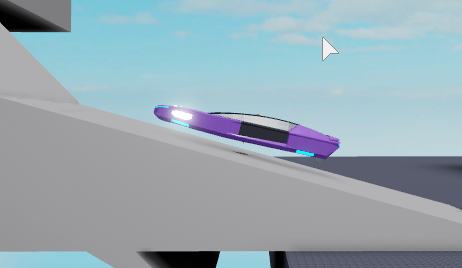# Hovercraft Changing Angles Frustrations

You’ve met car before:

So, I need to get onto this type of terrain:And this type:

But there is an issue, You see I use a global turn value:

``````local turn = 0
--later
if scal == 1 then --because this is in a change steering function
if turn + tSpeed.Value < math.rad(360) then
turn = turn + tSpeed.Value
else
turn = math.rad(360) - (turn + tSpeed.Value)
end
elseif scal == -1 then
if turn - tSpeed.Value > 0 then
turn = turn - tSpeed.Value
else
turn = (turn - tSpeed.Value) + math.rad(360)
end
end
``````

And how I calculate my new perpendicular angle is using the detected RightVector of the block its under:

``````local uAve = normAve:Cross(fRay.CFrame.RightVector).Unit
--norm average being the average of all the normals detected when raycasting downwards
``````

So, the turn global value gets messed up because it is only calculating from the new perpendicular vector we found.

Problem: When a new perpendicular vector is found, the turn angle is too big, so the car turns to a weird angle without the player telling it to move

What I want to do is make the turn value change according to the new perpendicular vector with relation to the last perpendicular vector. So if the angle between both vectors (on the x/z plane) is 90, then it subtracts 90 from the turn value.

Methods that didnt work:

1. Making an average of the right vectors from all raycast points on the craft and use the angle between the last and the new right vector
2. Getting the perpendicular vectors for the new and the last and determine the angle between both on the x/z plane

1 for some reason gets me -nan(ind) and 2 makes my car go upside down

Current Code
``````local function changeSteer(scal)
local backPerc

local stabFold = car.StabilityPoints

llAV = normAve
for _, poin in pairs(stabFold:GetChildren()) do
local h,_, fN = castRay(poin, (-1 * poin.CFrame.UpVector), 500000)
if h then
normAve = ((normAve.Unit + fN.Unit)/2).Unit
--lAngleVec = ((h.CFrame.RightVector + lAngleVec)/2).Unit
--print(h.CFrame.RightVector)
end
end

--print(normAve)
local fRay = castRay(stabFold.FPoint, (-1 * stabFold.FPoint.CFrame.UpVector), 500000)
if fRay == nil then
fRay = castRay(physRoot, (-1 * physRoot.CFrame.UpVector), 500000)
if fRay == nil then
fRay = castRay(stabFold.BPoint, (-1 * stabFold.BPoint.CFrame.UpVector), 500000)
end
end
local uAve = normAve:Cross(fRay.CFrame.RightVector).Unit
local xAve = llAV:Cross(fRay.CFrame.RightVector).Unit

local calcableAngleThing = Vector3.new(uAve.X, 0, uAve.Z)
local calcableAngleThing2 = Vector3.new(xAve.X, 0, xAve.Z)
--print(math.deg(math.acos(calcableAngleThing:Dot(calcableAngleThing2))))
turn = turn - math.acos(calcableAngleThing:Dot(calcableAngleThing2))

--print(math.deg(turn))

if scal == 1 then
turn = turn + tSpeed.Value
else
turn = math.rad(360) - (turn + tSpeed.Value)
end
elseif scal == -1 then
if turn - tSpeed.Value > 0 then
turn = turn - tSpeed.Value
else
turn = (turn - tSpeed.Value) + math.rad(360)
end
end
--backPerc changes
else
end

local dAve = -uAve
local yDif = dAve.Y - uAve.Y

local fVec = Vector3.new(0,0,-1)

--rotate it with the angle of turn
local px = (uAve.X * math.cos(turn)) - (uAve.Z * math.sin(turn))
local pz = (uAve.Z * math.cos(turn)) + (uAve.X * math.sin(turn))
uAve = Vector3.new(px, (uAve.Y + (yDif * backPerc)), pz)

--change that, broth
gyro.CFrame = CFrame.new(physRoot.Position, (physRoot.Position + uAve))
end
``````
1 Like

Why are you casting your rays so far down?

To ensure that it reaches a surface.
I solved my issue though. I basically optimized my code for the case.

Instead of using the surface’s right vector, I use the physics root.

``````local uAve = normAve:Cross(physRoot.CFrame.RightVector).Unit
``````